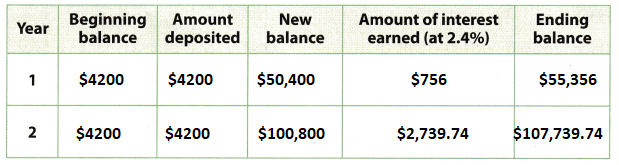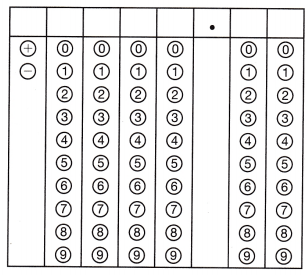Refer to our Texas Go Math Grade 8 Answer Key Pdf to score good marks in the exams. Test yourself by practicing the problems from Texas Go Math Grade 8 Module 16 Quiz Answer Key.

16.1 Repaying Loans

Dustin is taking out a loan for $2000 and wants to know how much money he will save by taking a 2-year loan at 14% interest instead of 20% interest. (Use an online calculator.) Question 1. What is the total repayment for the 20% loan?. Answer: Amount of loan =$2000
Interest Rate = 20%
Time Period = 2 year or 24 months
The total repayment for the 20% loan is $193.40Question 2. What is the total repayment for the 14% loan?. Answer: Amount of loan =$2000
Interest Rate = 14%
Time Period = 2 year or 24 months
The total repayment for the 14% loan is $156.52Question 3. How much can Dustin save? Answer: The total repayment for the 14% loan is$156.52
The total repayment for the 20% loan is $193.40$193.40 – $156.52 =$36.88
Thus Dustin save $36.88 16.2 Saving and Investing Question 4. Cecilia has$800 in an account earning 4.5% simple interest. How much more interest would her account earn in 7 years with annually compounded interest?
Given,
Cecilia has $800 in an account earning 4.5% simple interest. Initial amount =$800
Interest Rate = 4.5%
Period = 7 years
Total interest earned = $11,949.03 16.3 Analyzing Financial Situations Question 5. Byron has$250 in his savings account. He starts a new job next week and spends $300 on tickets to a sporting event to celebrate. Is his decision financially responsible or financially irresponsible? Explain. Answer: Irresponsible, because Byron does not have a steady source of income. 16.4 Estimating College Costs and Payments Question 6. At the beginning of each of the last two years, Alfonso put$4200 from his earnings as a part-time pizza delivery driver into a college savings account earning 2.4% interest compounded annually. Complete the table to determine how much money Alfonso has saved.Texas Go Math Grade 8 Module 16 Mixed Review Texas Test Prep Answer Key

Selected Response

Question 1.
Which interest rate and time period result in the lowest total loan repayment for a $4000 loan earning simple interest? (A) 3 years at 11% (B) 3 years at 13% (C) 4 years at 8% (D) 4 years at 11% Answer: (A) 3 years at 11% Formula for simple interest equal to SI = PTR/100 Here, P = actual money =$4000
T = time = 3 years.
R = interest rate = 11%
Simple interest for 3 years = $4000 × 3 × 11/100 =$1320
(B) 3 years at 13%
Formula for simple interest equal to SI = PTR/100
Here,
P = actual money = $4000 T = time = 3 years. R = interest rate = 13% Simple interest for 3 years =$4000 × 3 × 13/100 = $1560. (C) 4 years at 8% Formula for simple interest equal to SI = PTR/100 Here, P = actual money =$4000
T = time = 4 years.
R = interest rate = 8%
Simple interest for 4 years = $4000 × 4 × 8/100 =$1280
(D) 4 years at 11%
Formula for simple interest equal to SI = PTR/100
Here,
P = actual money = $4000 T = time = 4 years. R = interest rate = 11% Simple interest for 4 years =$4000 × 4 × 11/100 = $1760. The interest rate and time period result in the lowest total loan repayment for a$4000 loan = $1280 Option C is the correct answer. Question 2. Which equation represents a non proportional relationship? (A) y = -5x (B) y = 5x + 0 (C) y = $$\frac{1}{5}$$x (D) y = 5x – 5 Answer: y = $$\frac{1}{5}$$x Explanation: Let the ordered pair is (5, 5) y = $$\frac{1}{5}$$x 5 = $$\frac{1}{5}$$ × 5 5 = 1 Thus As y increases x decreases which means that the equation is nonproportional. So, option C is the correct answer. Question 3. Jemarcus starts with$1200 in a college savings account. His account earns interest at a rate of 1.8% compounded annually. How much money is in the account after 6 years?
(A) $1221.60 (B)$1265.97
(C) $1329.60 (D)$1335.57
Given that,
Jamarcus starts in a college savings account = $1200 The interest at the rate = 1.8% Formula for compound interest = A = P(1 + R/1000)n P = actual money =$1200
n = time = 6 years.
R = interest rate = 1.8%
Compound interest for 6 years = A = 1200(1 + 1.8/100)6
= 1200(1 + 0.018)6
= 1200(1.018)6
= 1200(1.1129)
The total money is in the account after 6 years= 1335.48
Option D is the correct answer.

Question 4.
Which equation relates x and y for the set of ordered pairs (4, 1), (8, 2), (12, 3)?
(A) y = $$\frac{1}{4}$$x
(B) y = 4x
(C) y = x – 3
(D) y = x – 9
(A) y = $$\frac{1}{4}$$x
(4, 1)
1 = $$\frac{1}{4}$$4
1 = 1
(8, 2)
2 = $$\frac{1}{4}$$8
(12, 3)
3 = $$\frac{1}{4}$$12
3 = 3
So, Option A is the correct answer.

Question 5.
Danielle received a gift card to a clothing store and uses it to buy a pair of jeans. Which payment method did she use?
(A) cash
(B) credit card
(C) debit card
(D) stored-value card
Given that,
Danielle received a gift card to a clothing store and he uses it to buy a pair of jeans.
The payment method he can use is a cash or credit card.
The credit card means it is a card issued by the bank to the holder to purchase goods or services.
Option A and B is the correct answer.

Question 6.
Ashley is considering attending the state university to obtain a 4-year bachelor’s degree. For one year, the tuition and fees are $9890, room and board are$8250, and books are $680. What will be the total of these costs over the 4 years of obtaining the degree? (A)$18,140
(B) $18,820 (C)$37,640
(D) $75,280 Answer: Given that, The total amount for 1 year Ashley’s tuition and fees =$9890.
The amount for 4 years of tuition and fees = $9890 × 4 =$39,560
The total amount for 1 year Ashley room and board = $8250. The amount for 4 years of room and board =$8250 × 4 = $33,000 The total amount for 1 year Ashley books =$680.
The total amount for 4 years of books = $680 × 4 =$2720
The total of these costs over the 4 years of obtaining the degree = $39,560 +$33,000 + \$2720 = 75,280.
Option D is the correct answer.

Question 7.
Triangle ABC, with vertices A(2, 3), 8(4, -5), arid C(6, 8), is reflected across the x-axis to form triangle A’B’C’. What are the coordinates of triangle A’B’C’?
(A) A'(2, -3), B'(4, 5), C'(6, -8)
(B) A'(-2, 3), B'(-4, -5), C'(-6, 8)
(C) A'(-2, -3), B'(-4, 5), C'(-6, -8)
(D) A'(2, -3), B'(4, -11), C'(6, 2)
The reflection of the point (x,y) on the x- axis is (x,-y).
The vertices A(2, 3), 8(4, -5), and C(6, 8) reflected on the x axis are A'(2, -3), B'(4, 5), C'(6, -8).
Option A is the correct answer.

Gridded Response

Question 8.
A cone-shaped cup has a height of 3 inches and a volume of 9 cubic inches. What is the length in inches of the diameter of the cone? Use 3.14 for π. Round your answer to the nearest hundredth.Given that,
Height of the cone-shaped cup = 3 inches.
The volume of the cone-shaped cup = 9 inches.
The formula for the radius of the cone = square root of (3× V/πh)
= square root of (3 × 9/3.14 × 3)
= square root of (27/9.42)
= square root of (2.86)
= 1.691
Therefore the length of the cone = 2πr
= 2 × 3.14 × 1.691
= 10.61
The length of the diameter of a cone-shaped cup is 10.61.

Scroll to Top